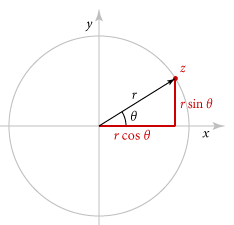ROS Resources: Documentation | Support | Discussion Forum | Index | Service Status | ros @ Robotics Stack Exchange

# need explanation on sensor_msgs/LaserScan.msgHi, i am new in ROS, the message Definition of a LaserScan.msg is as follows:-

std_msgs/Header header
float32 angle_min
float32 angle_max
float32 angle_increment
float32 time_increment
float32 scan_time
float32 range_min
float32 range_max
float32[] ranges
float32[] intensities


i want to know the what information these variable holds and how can i manipulate them to extract the distance from an obstacle.

edit retag close merge delete

Sort by » oldest newest most votedDoc: sensor_msgs..LaserScan has explanation for all the variables.

Looking at the code Publish_a_LaserScan_Message will gives good picture of variables.

laser scanner view of hokuyo URG-04LX-UG01 laser scannerred,green are x,y-axes

Values for above scanner

    angle_min= -135 * (pi/180); //angle correspond to FIRST beam in scan ( in rad)
angle_max= 135 * (pi/180); //angle correspond to LAST beam in scan ( in rad)
angle_increment =0.25 * (pi/180); // Angular resolution i.e angle between 2 beams

// lets assume sensor gives 50 scans per second. i.e every 20 milli seconds 1 scan with 1081 beams.
// Each beam is measured in  (20 ms/ 1081 ) ~ = 0.0185 ms

time_increment  = (1 / 50) / (1081);

scan_time = ;   // scan is collected at which time
range_min =0 ; // in meters
range_max = 20; // scan can measure upto this range
// ranges is array of 1081 floats for each laser beam
ranges = //distance measure corresponds to angle -135 deg
ranges = //distance measure corresponds to angle -134.75 deg
.
.
.
ranges = //distance measure corresponds to angle +135 deg

//To understand Intensities
//if a laser beam hits reflective surface like glass it will have intensity 1.
//And if beam hit some surface which absorbs laser , then intensity is zero.
//Middle values are different surfaces in between.


Header header            # timestamp in the header is the acquisition time of
# the first ray in the scan.
#
# in frame frame_id, angles are measured around
# the positive Z axis (counterclockwise, if Z is up)
# with zero angle being forward along the x axis

float32 angle_min        # start angle of the scan [rad]
float32 angle_max        # end angle of the scan [rad]
float32 angle_increment  # angular distance between measurements [rad]

float32 time_increment   # time between measurements [seconds] - if your scanner
# is moving, this will be used in interpolating position
# of 3d points
float32 scan_time        # time between scans [seconds]

float32 range_min        # minimum range value [m]
float32 range_max        # maximum range value [m]

float32[] ranges         # range data [m] (Note: values < range_min or > range_max should be discarded)
float32[] intensities    # intensity data [device-specific units].  If your
# device does not provide intensities, please leave
# the array empty.


Obstacle distance calculation is easyif r, θ are range and angle from one scan laser scan obstacle is at (x,y) = ( rcosθ, rsinθ ) in laser frame.

To make sense about θ , here imagine your laser has 180 deg view and now looking towards x-axis and ray along postive y-axis is +90 degress and negative y-axis is -90 degree.

agnle_min = -90
agnle_max = 90

more

hi @bvbdort, i have gone through Doc: sensor_msgs..LaserScan, but i could not properly understand what those variable holds in them eg range_max, ranges, intensities ect, that's why I've posted this question for a brief explanation.

@Aarif , Max reading depends on what laser you are using , it can be 5m or 30m or different depending on laser you are using. Min reading is zero. To understand Intensities , if a laser beam hits reflective surface like glass it will have intenstiy 1.

And if beam hit some suface which absorbs laser , then intensity is zero. and middle values are different sufaces with relective nature.

Please let me know what exactly you are trying to understand.

thank you so much @bvbdort, can you please explain me about all the variables, thanks in advance. :)

hi @bvbdort, i have a P3AT spawned in Usarsim Simulator, i want measure its distance with wall or any other obstacle, for this i need to subscribe laser_scan topic which will give the data in the laser_scan.msg format which has these variables, thats why i wanted to know about these variables. Thnks

if you are looking for lms200 values specifically, go through lms200 data sheet

Thank you very much @bvbdort, now i've understand the things. :)

1

Wow. (pi/3.14) Won't they be just 1 and should be eliminated instead.